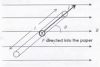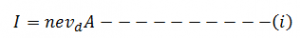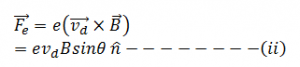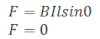# Force On Current Carrying Conductor On Magnetic FieldA conductor consists of large no. of free electrons. Current on the conductor means drifting of such a free electron in any fixed direction due to the motion of such a free electron each electron experience magnetic force and hence conductor itself experience magnetic force. So, when a current carrying conductor is placed in a uniform magnetic field it experience force.

Consider a conductor having length l, cross-section area A in a uniform magnetic field. If n be the number of electrons per unit volume (electron density), vd be the drift velocity of electron having electronic charge e then the current on the conductor is:Due to the motion of electron on magnetic field, each electron experience Lorent’s magnetic force.So the force experienced by the conductor is perpendicular to the plane containing l and B.

when the current carrying conductor is placed perpendicular to the magnetic field, magnetic field produces maximum force on the conductor.

Case-II:
if θ = 0° or 180°when the current carrying conductor is placed parallel or anti parallel with magnetic field then magnetic field produces no force on the conductor.